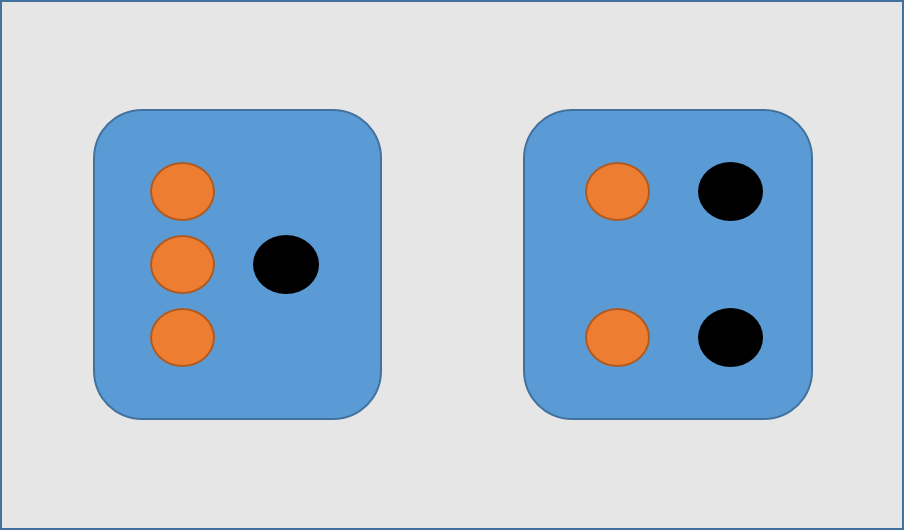# 一个例子搞清楚（先验分布/后验分布/似然估计）

## 一个例子搞清楚（先验分布/后验分布/似然估计）

preface：

• 无论是《通信原理》、《信息论》、《信道编码》还是《概率与统计理论》，或者在现在流行的《模式识别》《Machine Learning》中总会遇到这么几个概念：先验分布/后验分布/似然估计
• 如果大家不熟悉这几个词，相信大家熟知贝叶斯公式，该公式涉及到了以上几个概念。但是学完本科课程，也会算题，就是在实际情境中总感觉理不清这几个概念的关系，最近上课老被老师讲的先验、后验搞得晕头转向。因此，如果您和我遇到类似的囧事，这篇文章很适合您。
• 声明：本文主要内容修改整理于知乎回答1

• 一个隔壁小哥的故事
• 故事中的因果和三个概念
• 贝叶斯公式的角色
• 最大似然估计和贝叶斯的关系

### 三个概念之后验（知果求因）

P ( 交 通 方 式 ∣ 花 费 的 时 间 ) P( 交通方式 | 花费的时间 )

P ( 因 ∣ 果 ) P( 因 | 果 )

P ( θ ∣ x ) P( \theta | x )

[解释]：看到这里估计大家很奇怪为什么要用 x x θ \theta 这样的字母表示，而不是熟悉的 x x y y 。这样表示自然是有原因的。在这里大家只需要先暂时记住 θ \theta 代表 x x 代表，后面的贝叶斯我们将会具体介绍这些字母的含义。

### 三个概念之先验概率（由历史求因）

P ( 交 通 方 式 ) P(交通方式)

P ( 因 ) P( 因 )

P ( θ ) P(\theta)

### 三个概念之似然估计（由因求果）

P ( 时 间 ∣ 交 通 方 式 ) P(时间|交通方式)

P ( 果 ∣ 因 ) P( 果|因 )

P ( x ∣ θ ) P( x|\theta )

### 贝叶斯公式

P ( A ∣ B ) = P ( B ∣ A ) ∗ P ( A ) P ( B ) P( A|B )=\frac{P(B|A) *P(A)}{P(B)}

P ( θ ∣ x ) = P ( x ∣ θ ) ∗ P ( θ ) P ( x ) P( \theta|x )=\frac{P(x|\theta) *P(\theta)}{P(x)}

[注] P ( x ) P(x) e v i d e n c e evidence 。隔壁小哥去公园很多次，忽略交通方式是什么，只统计每次到达公园的时间 x x ，于是得到了一组时间的概率分布。这种不考虑原因，只看结果的概率分布即 e v i d e n c e evidence ，它也称为样本发生的概率分布的证据

e v i d e n c e evidence 在故事中如下表示：
P ( 时 间 ) P(时间)
P ( 果 ) P(果)

### 深入贝叶斯推断

(1) 现在小哥将随机选择一个箱子，从中摸出一颗糖。请问小哥选择一号箱子的概率有多大？
(2) 现在小哥将随机选择一个箱子，从中摸出一颗糖发现是水果糖。请问这颗水果糖来自一号箱子的概率有多大？P ( θ ∣ x ) = P ( x ∣ θ ) ∗ P ( θ ) P ( x ) P( \theta|x )=\frac{P(x|\theta) *P(\theta)}{P(x)}
[解释]：其中 x x 是观测得到的结果数据 P ( x ) P(x) 是观测结果数据的概率分布。如下表：

x x 水果糖巧克力糖
P ( x ) P(x) 5/83/8

[解释]：其中 θ \theta 决定观测结果数据分布的参数 P ( θ ) P(\theta) 是先验概率，没有观测数据的支持下 θ \theta 发生的概率。如下表：

θ \theta 一号箱二号箱
P ( θ ) P(\theta) 1/21/2

[解释] P ( θ ∣ x ) P(\theta|x) 是后验概率，有观测数据的支持下 θ \theta 发生的概率。在上面的故事中第二问是小哥随机选择一个箱子，从中摸出一颗糖发现是水果糖。这颗水果糖来自一号箱子的概率就是后验概率：
P ( θ = 一 号 箱 ∣ x = 水 果 糖 ) P(\theta =一号箱 | x = 水果糖)
[解释] P ( x ∣ θ ) P(x|\theta) 是似然函数，给定某参数 θ \theta 结果数据的概率分布

P ( x = 水 果 糖 ) = ∑ i P ( x = 水 果 糖 ∣ θ = i 号 箱 ) P ( θ = i ) P(x=水果糖) = \sum_{i}P( x = 水果糖 | \theta =i 号箱)P(\theta = i)

(1) 现在小哥将随机选择一个箱子，从中摸出一颗糖。请问小哥选择一号箱子的概率。根据明显的先验知识我们就可以知道
P ( θ = 一 号 箱 ) = 1 / 2 P(\theta =一号箱) =1/2
(2) 现在小哥将随机选择一个箱子，从中摸出一颗糖发现是水果糖。请问这颗水果糖来自一号箱子的概率。后验概率为
P ( θ = 1 ∣ x = 水 果 ) = P ( x = 水 果 ∣ θ = 1 ) ∗ P ( θ = 1 ) P ( x = 水 果 ) P( \theta =1|x=水果 )=\frac{P(x=水果|\theta =1) *P(\theta =1)}{P(x=水果)}
P ( θ = 1 ∣ x = 水 果 ) = P ( x = 水 果 ∣ θ = 1 ) ∗ P ( θ = 1 ) ∑ i P ( x = 水 果 糖 ∣ θ = i 号 箱 ) P ( θ = i ) P( \theta =1|x=水果 )=\frac{P(x=水果|\theta =1) *P(\theta =1)}{ \sum_{i}P( x = 水果糖 | \theta =i 号箱)P(\theta = i)}
P ( θ = 1 ∣ x = 水 果 ) = ( 3 / 4 ) ∗ ( 1 / 2 ) ( 3 / 4 ) ∗ ( 1 / 2 ) + ( 2 / 4 ) ∗ ( 1 / 2 ) P( \theta =1|x=水果 )=\frac{(3/4) *(1/2)}{ (3/4) *(1/2)+(2/4) *(1/2)}
P ( θ = 1 ∣ x = 水 果 ) = 3 / 5 P( \theta =1|x=水果 )=3/5

### MAP/ML/贝叶斯估计

• 最大似然估计（ML，Maximum Likelihood）可以估计模型的参数。其目标是找出一组参数 θ \theta ，使得模型产生出观测数据 x x 的概率最大：

a r g m a x θ P ( x ∣ θ ) \underset{\theta}{argmax} P(x|\theta)

• 假如这个参数有一个先验概率，那么参数该怎么估计呢？这就是MAP要考虑的问题。 最大后验估计（MAP－Maxaposterior）。MAP优化的是一个后验概率，即给定了观测值后使概率最大：

a r g m a x θ P ( θ ∣ x ) = a r g m a x θ P ( x ∣ θ ) ∗ P ( θ ) P ( x ) \underset{\theta}{argmax} P(\theta|x)=\underset{\theta}{argmax} \frac{P(x|\theta) *P(\theta)}{P(x)}

a r g m a x θ P ( θ ∣ x ) = a r g m a x θ P ( x ∣ θ ) ∗ P ( θ ) \underset{\theta}{argmax} P(\theta|x)=\underset{\theta}{argmax} P(x|\theta) *P(\theta)

P o s t e r i o r ∝ ( L i k e l i h o o d ∗ P r i o r ) Posterior∝(Likelihood∗Prior)
P ( x ) P(x) 相当于是一个归一化项，整个公式就表示为：后验概率 正比于 先验概率 ∗ * 似然函数。

• 前两种都是假设参数是个确定值，但贝叶斯估计假设参数是个随机数
贝叶斯估计，假定把待估计的参数看成是符合某种先验概率分布的随机变量，而不是确定数值。在样本分布上，计算参数所有可能的情况，并通过计算参数的期望，得到后验概率密度。

1. 这里是 脚注内容. ↩︎

09-28468910-231015
10-062455
07-271348
11-104万+
07-28756
07-115372
06-072651
05-282108
05-146万+
11-221万+
06-245149
11-241万+
07-231054
09-127523
08-05446
12-151989
07-068579
03-213万+
09-192180